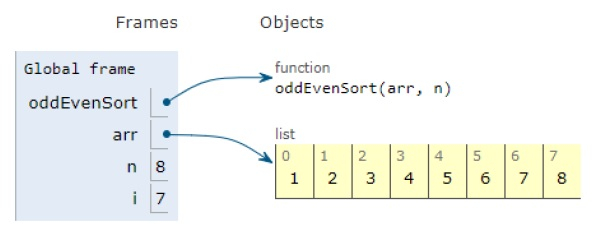# Python Program for Odd-Even Sort / Brick Sort

Problem statement − We are given an array, we need to sort it using brick sort.

Here we have two phases: Odd and Even Phase. In the odd phase, bubble sort is performed on odd indexed elements and in the even phase, bubble sort is performed on even indexed elements.

Now let’s observe the solution in the implementation below−

## Example

def oddEvenSort(arr, n):
# flag
isSorted = 0
while isSorted == 0:
isSorted = 1
temp = 0
for i in range(1, n-1, 2):
if arr[i] > arr[i+1]:
arr[i], arr[i+1] = arr[i+1], arr[i]
isSorted = 0
for i in range(0, n-1, 2):
if arr[i] > arr[i+1]:
arr[i], arr[i+1] = arr[i+1], arr[i]
isSorted = 0
return
arr = [1,4,2,3,6,5,8,7]
n = len(arr)
oddEvenSort(arr, n)
print(“Sorted sequence is:”)
for i in range(0, n):
print(arr[i], end =" ")

## Output

Sorted sequence is:
1 2 3 4 5 6 7 8All the variables are declared in the local scope and their references are seen in the figure above.

## Conclusion

In this article, we have learned about how we can make a Python Program for Odd-Even Sort /Brick Sort